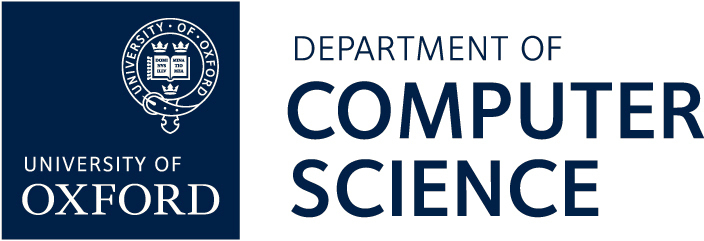# Oxford Quantum Talks Archive

Computational Complexity of Geometric Quantum Logic

Martin Ziegler, Technical University of Darmstadt
Quantum Physics and Logic 2010, May 2010, University of Oxford

We propose a new approach towards the question of whether the equational theory of ortholattices related to Quantum Mechanics is decidable or not: By determining the growth of the algorithmic complexity of the word problem over d-dimensional Hilbert lattices for d=1,2,3,... In case d=1, the (complement of the) word problem amounts to the Boolean satisfiability problem underlying the millennium question `P=NP' We show the case d=2 to be NP-complete as well. For fixed d>=3 and building on Hagge et.al (2005,2007,2009), we reveal quantum satisfiability as polytime-equivalent to the real feasibility of a multivariate quartic polynomial equation: a problem well-known complete for the counterpart of NP in the Blum-Shub-Smale model of computation lying (probably strictly) between classical NP and PSPACE. We finally address the problem over indefinite finite dimensions.

[video] [streaming video]